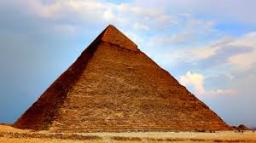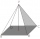Find the volume and surface of a regular quadrilateral pyramid if the bottom edge is 45 cm long and the pyramid height is 7 cm.

V =  4725 cm3
S =  4145.7369 cm2

### Step-by-step explanation:Did you find an error or inaccuracy? Feel free to write us. Thank you!Tips to related online calculators
Tip: Our volume units converter will help you with the conversion of volume units.
Pythagorean theorem is the base for the right triangle calculator.

#### You need to know the following knowledge to solve this word math problem:

We encourage you to watch this tutorial video on this math problem:

## Related math problems and questions:

• Find theFind the surface area of a regular quadrilateral pyramid which has a volume of 24 dm3 and a height of 45 cm.What is the volume of a regular quadrilateral pyramid if the edge of the base is 8 cm long and the height of the side wall is 5 cm?What is the volume of a regular quadrilateral pyramid if its surface is 576 cm2 and the base edge is 16 cm?Find the height and surface of a regular quadrilateral pyramid with a base edge a = 8cm and a wall height w = 10cm. Sketch a picture.
• Pyramid 4sidesCalculate the volume and the surface of a regular quadrangular pyramid when the edge of the base is 4 cm long and the height of the pyramid is 7 cm.
• Hexagonal pyramidFind the area of a shell of the regular hexagonal pyramid, if you know that its base edge is 5 cm long and the height of this pyramid is 10 cm.
• Hexagonal pyramidCalculate the volume and the surface of a regular hexagonal pyramid with a base edge length of 3 cm and a height of 5 cm.
• The regularThe regular quadrilateral pyramid has a volume of 24 dm3 and a height of 45 cm. Calculate its surface.We have a regular quadrilateral pyramid with a base edge a = 10 cm and a height v = 7 cm. Calculate 1/base content 2/casing content 3/pyramid surface 4/volume of the pyramid
• Truncated pyramidFind the volume and surface area of a regular quadrilateral truncated pyramid if base lengths a1 = 17 cm, a2 = 5 cm, height v = 8 cm.
• Triangular pyramidDetermine the volume and surface area of a regular triangular pyramid having a base edge a=20 cm and a lateral edge b = 35 cm
• Pyramid heightFind the volume of a regular triangular pyramid with edge length a = 12cm and pyramid height h = 20cm.
• Hexagon cut pyramidCalculate the volume of a regular 6-sided cut pyramid if the bottom edge is 30 cm, the top edge is 12 cm, and the side edge length is 41 cm.
• Triangular pyramidCalculate the volume and surface area of a regular triangular pyramid with height equal to the base edge 10 cm length.
• Tetrahedral pyramidA regular tetrahedral pyramid is given. Base edge length a = 6.5 cm, side edge s = 7.5 cm. Calculate the volume and the area of its face (side area).
• Pyramid four sidesIn a regular tetrahedral pyramid is a body height of 38 cm and a wall height of 42 cm. Calculate the surface area of the pyramid; the result round to square centimeters.
• Regular hexagonal pyramidCalculate the height of a regular hexagonal pyramid with a base edge of 5 cm and a wall height of w = 20cm. Sketch a picture.